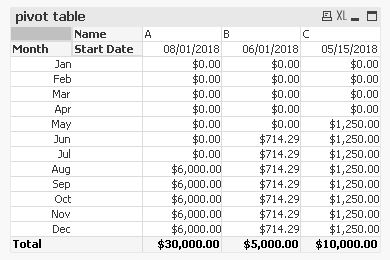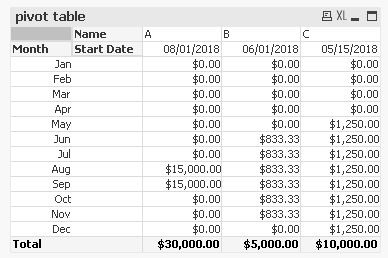QlikView Scripting

Discussion Board for collaboration on QlikView Scripting.

Announcements
QlikWorld 2020: Join us May 11 - 14, 2020 in Phoenix, AZ. Register early and save \$400. Learn More
Highlighted
New Contributor II

Load in for each loop that iterates over months

Hello Qlik Community,

I have a spreadsheet that has a name, start date, a yearly labor cost. What I want to do is evenly divide the labor cost by the 12 months in a year - start date, in other words, the remaining months in the year. For example, if the start date was June 15, and the labor cost for the year was 1000 dollars, then the labor cost for June would be yearly labor cost / (12 - 6(June) + 1), for July labor cost would be yearly labor cost / (12 - 6(June) + 1), etc. The labor cost for the months before start date would just be 0. My load script is executing with no errors but the data is not loading correctly. There are the correct number of rows being loaded (1080) but direct labor is always 0.

Thank you in advance,

Zach

for each mon in 'January', 'February', 'March', 'April', 'May', 'June', 'July', 'August', 'September', 'October', 'November', 'December'

"Name",

Date("Start Date",'MM DD YY') as "Start Date",

"Direct Labor",

'\$(mon)' as Month,

if('\$(mon)' > num(month("Award Date")), 0, "Direct Labor" / (12 - num(month("Award Date")) + 1)) as "Direct Labor",

"Base Years",

"Option Years",

"2019 Performance Months"

FROM [lib://AttachedFiles/Example.xlsx]

(ooxml, embedded labels, table is [Direct Labor]);

next

1 Solution

Accepted SolutionsMVP

Re: Load in for each loop that iterates over months

Hi,

I guess you're looking for something like this?:table1:

Date([Start Date]) as [Start Date],

Money([2019 NTC Direct Labor]) as [2019 NTC Direct Labor],

Month(Date#(IterNo(),'M')) as Month,

Money(If(IterNo()>=Month([Start Date]),[2019 NTC Direct Labor]/(13-Month([Start Date])),0)) as [Direct Labor]

FROM [https://community.qlik.com/servlet/JiveServlet/download/1463115-320502/Example.xlsx] (ooxml, embedded labels, table is Sheet1)

While IterNo()<=12;

hope this helps

regards

Marco

7 Replies
New Contributor II

Re: Load in for each loop that iterates over months

Using your example (There is no column in there called "Award Date", by the way) I came up with this:

(No loop required)

SET MonthNumbers='1;2;3;4;5;6;7;8;9;10;11;12';

MonthDivide:

if(MonthNum>=StartNum,Round((DirectLaborTotal / divisor),0.01),0) as "DirectLabor"

;

Name,

Date("Start Date") as "Start Date",

Num(Month(Date("Start Date"))) as StartNum,

Num(SubField('\$(MonthNumbers)', ';')) as MonthNum,

12 - (num(month("Start Date")-1)) as divisor,

Num("2019 NTC Direct Labor") as DirectLaborTotal

FROM [lib://Desktop/Example.xlsx]

(ooxml, embedded labels, table is Sheet1)

WHERE ISNull(Name) = 0;MVP

Re: Load in for each loop that iterates over months

Hi,

I guess you're looking for something like this?:table1:

Date([Start Date]) as [Start Date],

Money([2019 NTC Direct Labor]) as [2019 NTC Direct Labor],

Month(Date#(IterNo(),'M')) as Month,

Money(If(IterNo()>=Month([Start Date]),[2019 NTC Direct Labor]/(13-Month([Start Date])),0)) as [Direct Labor]

FROM [https://community.qlik.com/servlet/JiveServlet/download/1463115-320502/Example.xlsx] (ooxml, embedded labels, table is Sheet1)

While IterNo()<=12;

hope this helps

regards

Marco

New Contributor II

Re: Load in for each loop that iterates over months

Marco,

Perfect!

That is exactly what I am looking for, thank you very much. Quick follow on, say I had another field in my spreadsheet, numMonths, which specified the number of months labor costs should be split across. Say start in August and duration was two months, the total labor cost should only be split across August and September. I need to add something to the if statement, so far I have something like this but I cannot seem to get it:

Money(If(IterNo()>=Month([Start Date]) and '\$(Month)' <= Month([StartDate]) + Month(Date#([numMonths], 'M')),[2019 NTC Direct Labor]/numMonths,0)) as [Direct Labor]

Thanks for your help.

Zach

New Contributor II

Re: Load in for each loop that iterates over months

Thank you Russ, also exactly what I was looking for. Apologize about the field confusion. Had to change an internal sheet.

Thanks,

ZachMVP

Re: Load in for each loop that iterates over months

Nice to hear that it works for you.

thanks

regards

Marco

New Contributor II

Re: Load in for each loop that iterates over months

I believe I have just done so (press mark correct answer.) Any insight into follow up question regarding changing the if condition?

Thanks,

ZachMVP

Re: Load in for each loop that iterates over months

Hi,

thanks.

adding some numMonths values to your example, one solution could be:table1:

Date([Start Date]) as [Start Date],

Money([2019 NTC Direct Labor]) as [2019 NTC Direct Labor],

Month(Date#(IterNo(),'M')) as Month,

Money(If(IterNo()>=Month([Start Date]) and IterNo()<Month([Start Date])+numMonths,[2019 NTC Direct Labor]/numMonths,0)) as [Direct Labor]

INLINE [

Name, Start Date, 2019 NTC Direct Labor, numMonths

A, 43313, 30000, 2

B, 43252,  5000, 6

C, 43235, 10000, 8

]

While IterNo()<=12;

hope this helps

regards

Marco